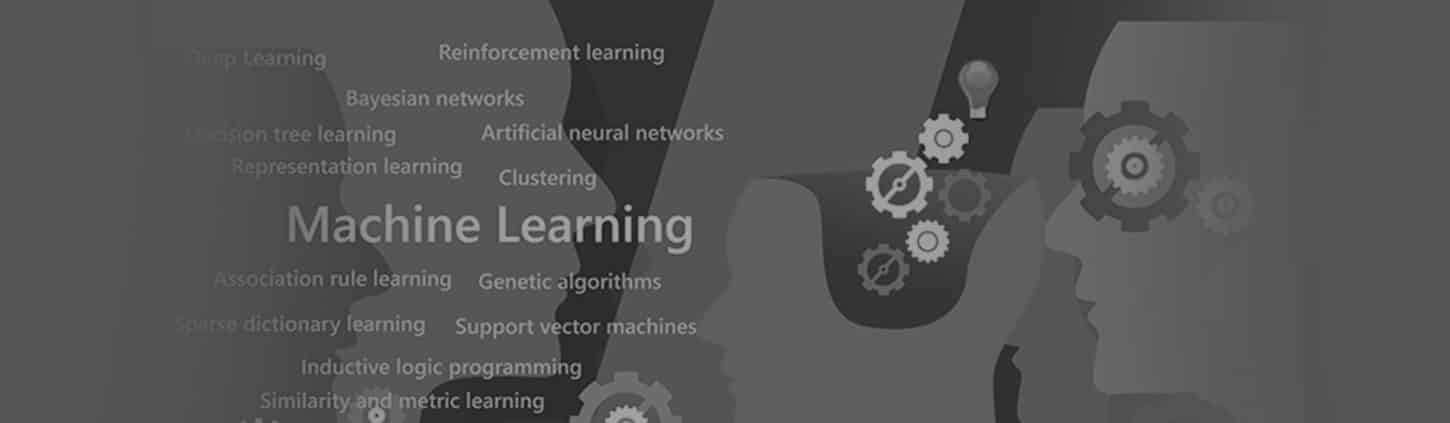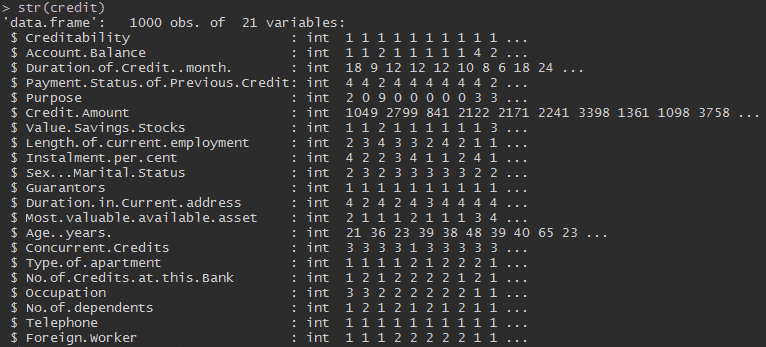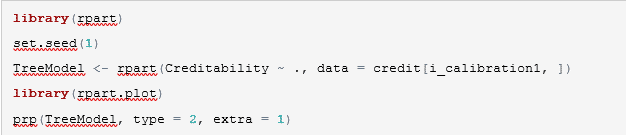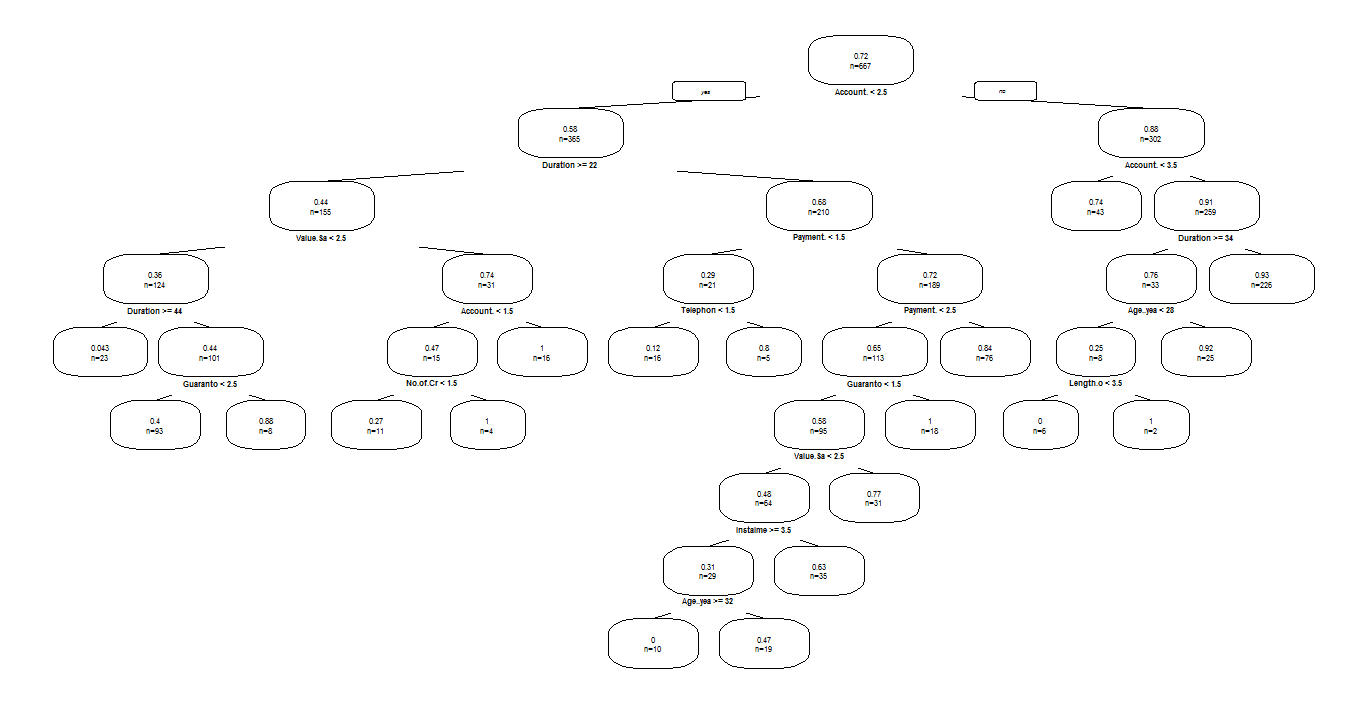# Supervised Machine Learning

## Introduction

Machine Learning is a core subarea of ‘’Artificial Intelligence’’, which learns from an existing model, results, and observations. We can define it as a subfield of computer science that gives computers the ability to learn without being explicitly programmed.”

More in detail, machine learning is a set of techniques or algorithms which are used to program computers and make decisions automatically and more accurately.

#### How does “Machine Learning” make decisions?

It makes decisions by analyzing (or learning) pattern in the past data and applying it to the future data. There can be different forms of decisions such as predictions of the customer behavior, financial decisions – stock price prediction, fraud detection and much more.

#### Types of Machine Learning Algorithm:

Majorly there are three different types of machine learning algorithm:

• Supervised Machine Learning
• Un-Supervised Machine Learning
• Reinforcement Machine Learning

#### Supervised Machine Learning(SML)

In this blog, precisely we’ll talk about the supervised machine learning, which is one of the most commonly used types of machine learning algorithms.

The majority of practical machine learning uses supervised learning. In Supervised machine learning algorithms, we have both input(X) and output(Y) variables and the algorithm generates a function that predicts the output(Y) based on given input(X) variables. It is called ‘supervised’ because the algorithm learns in a supervised manner. This learning process iterates over the training data until the model achieves an expected or closest to the expected result.

Supervised learning problems can be further divided into two parts:

• Regression: A supervised problem is said to be regression problem when the output variable is a continuous value such as “weight”, “height” or “dollars.”
• Classification: It is said to be a classification problem when the output variable is a discrete (or category) such as “male” and “female” or “disease” and “no disease.”

### Application of Supervised Learning:

#### Classification Method – Decision Tree

Here, I am using the “German Credit” data for the sake of understanding, having 1000 number of records and 21 columns, let’s see what information we can get from this data using predictive analytic techniques?Now, we have the overview of the credit data and having the basic idea about the credit data.

Let’s start working with a supervised learning method – “Decision Tree” (DT) and for that, I am going to use R tool. These are the classification models that partition data into subsets based on categories of input variables.Initially, we decide the target variable i.e. Creditability, later looking the impact of other variables on target variable and we can treat them accordingly.

Below, the DT plot from which it can easily identify the different levels and nodes of DT. The classification criteria for the different levels are calculated with the help of entropy.

Decision – Tree:The multi-layered decision tree above clearly shows the distribution of different levels based on entropy value. The decision tree gives us the decision level idea of the data where we can take the complex business decision and knowing the profitable customers as well.

#### Some popular examples of supervised machine learning algorithms are:

• Linear regression for regression problems.
• Random forest for classification and regression problems.
• Support vector machines for classification problems.

#### What Else Can We Do with Supervised Machine Learning?

• Speech Recognition
• Face detection
• Fraudulent activities detection
• Social network analysis to define groups of friends

Conclusion:

Supervised learning is just getting good with every coming day. Machine learning can make the impossible things possible with the higher accuracy rate.

In short, Machine learning is considered as one of the most trending technological methodologies for better innovations.

For more insights on Supervised Machine Learning feel free to get in touch with us through sales@bistasolutions.com, you can also write your feedback on how this blog has helped you at feedback@bistasolutions.com.